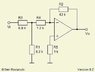# Ideal Op-Amp question

spoonjabba
Derive a symbolic expression (i.e., a formula containing symbols R1, R2, ..., etc., andarithmetic operators instead of numerical values) for the voltage gain of the amplifier

What I am stuck at is the two nodes on each side of R4

I initially disregarded the node to the right of R4, which was wrong. since there is a virtual sc at the input of the op amp terminals what would i consider the node on the right of r4?

#### Attachments

•IdOp_P4_Diagram.jpg
7.2 KB · Views: 258
Last edited:

Homework Helper
Gold Member
Since no current is flowinginto either -/+ terminal as well, the voltage at the + terminal is 0V.

Homework Helper
Gold Member
Derive a symbolic expression (i.e., a formula containing symbols R1, R2, ..., etc., andarithmetic operators instead of numerical values) for the voltage gain of the amplifier

What I am stuck at is the two nodes on each side of R4

I initially disregarded the node to the right of R4, which was wrong. since there is a virtual sc at the input of the op amp terminals what would i consider the node on the right of r4?

You just said to consider the node on the right of R4 to be a sc which I assume must mean short circuit or, as we call it, ground. The problem?